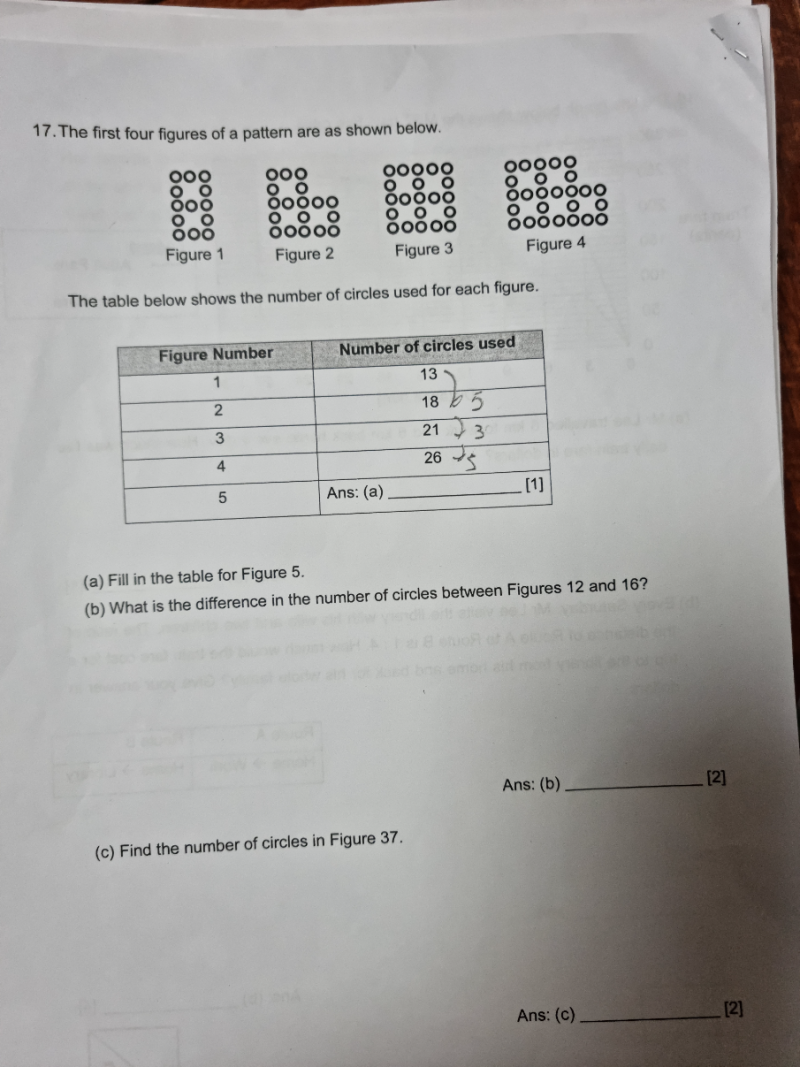# QuestionThank you

Observing how the figures tile will help answer the first question.

Fig 1 13

fig 2 18

fig 3 21

fig 4 26

(a) fig 5 29

The table will help to answer the second part as the difference between two figures are always either 5 or 3

If fig 12 is fig 1, fig 16 is fig 5. The difference between fig 1 and fig 5 are

(b) 29 – 13 = 16

The third part requires us to identify a pattern and code that pattern.

fig 1. (2 +1) x 5 – (2 x (2/2)) = 13

fig 2. (2 + 2) x 5 – (2 x (2/2)) = 18

fig 3. (2 + 3) x 5 – (2 x (4/2)) = 21

fig 4. (2 + 4) x 5 – (2 x (4/2)) = 26

fig 5. (2 + 5) x 5 – (2 x (6/2)) = 29

Notice the left changing number equals the fig number. The right changing number is the fig number divided by 2 when it is even. For example when fig number is 4, the right changing number is 4/2 = 2.

(c) fig 37. (2 + 37) x 5 – (2 x (38/2)) = 157

0 Replies 1 Like

Fig               Circles

1                    13                 (+5)

2                    18                (+3)

3                    21                 (+5)

4                    26                (+3)

5                 26+3 = 29

(a) Hence Figure 5 has 29 circles.

(b) There are 2 sequences here in the pattern, one odd and one even.

Even term sequence:  18, 26, 34….  first term = 18, difference = 8

pth term = 18+ (p-1) x 8     where n = 2p (even number figure)

Odd term sequence:  13, 21, 29……     first term = 13, difference = 8

rth term = 13 + (r-1) x 8   where n = 2r-1 (odd number figure)

Part (b) refers to Fig 12 and 16 and both are even terms,

So  n = 12 =>  p = 12/2 = 6,   So Fig 12 = p6 = 18 + (6-1)8 = 58

n=16   => p = 16/2 = 8,    So Fig 16 = p8 =18 + (8-1)8 = 74

Hence difference in number of circles between Fig 16 and Fig 12 = 74-58 =16

(c) Fig 37 is an odd term

So n = 37,   2r= 37+1 = 38  => r = 19,  So Fig 37 = r19 = 13 + (19-1) 8 = 157

PS:  The nth term of an arithmetic sequence is given by :

nth term = a + (n – 1)d           where a= first term, d = constant difference between terms

0 Replies 1 Like